International Journal of Mechanical Engineering and Applications
Volume 4, Issue 2, April 2016, Pages: 65-70

Analysis of Aeroelastic Stability of Long Straight Wing with Store System

Yan-Ping Xiao1, 2, *, Yi-Ren Yang1

1Southwest Jiaotong University, Chengdu, Sichuan, China

2Civil Aviation Flight University of China, Guanghan, Sichuan, China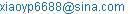(Yan-Ping Xiao)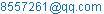(Yi-Ren Yang)

*Corresponding author

Yan-Ping Xiao, Yi-Ren Yang. Analysis of Aeroelastic Stability of Long Straight Wing with Store System. International Journal of Mechanical Engineering and Applications.Vol. 4, No. 2, 2016, pp. 65-70. doi: 10.11648/j.ijmea.20160402.15

Received: March 28, 2016; Accepted: April 7, 2016; Published: April 19, 2016

Abstract: The aeroelastic equations of long straight wing with store system are developed in this paper by applying the Hamilton’s Principle. The dynamical model takes the store as an independent degree of freedom and considers the geometric nonlinearity of wing. The system dynamics is numerically simulated by using the Galerkin’s method. Results show that the critical flutter speed becomes largest when the store locates at wingtip and around 40% half chord before the elastic axis. The critical flutter speed will decrease as the wing-store joint rigidity decreases. On the other hand, it is shown that sudden change of flutter frequency might occur when the wing-store joint rigidity increases. Moreover, numerical results indicate buckling boundary is independent of store parameters. When the joint rigidity is relatively small, the system flutter occurs first. When the joint rigidity is relatively large, buckling occurs first. With the presence of geometric nonlinearity and increasing flow speed, the system behavior will evolve from limit cycle oscillation, to quasi-periodical motion and eventually to chaos.

Keywords: Wing, Store, Stability, Geometric Nonlinearity, Flutter, Buckling

Contents

1. Introduction

Wing store can be found in both military and civilian aircrafts. The mass, location and wing-store joint rigidity of the store can all influence the aeroelastic stability of the wing-store system. Intensive researches have been conducted to study the dynamical behavior of wing-store systems. Yang Yiren  analyzed the limit cycle flutter of the wing with store system using KBM method. Yang Zhichun  studied flutter speed using two-dimensional wing-store system and the effect of pitch joint rigidity on the flutter boundary was analyzed. Zhang Jiang  studied the aeroelastic stability of high-aspect-ratio flexible wings subject to a lateral follower force. The results show the lateral follower force could stabilize the wing. Wang Ganglin  investigated the vibration and flutter characteristics of the wing with the steady aerodynamics under different angle of attack and different engine thrust. Xu Jun  considered the in-plane bending of high aspect ratio wing and analyzed the effect of store mass and store moment of inertia on the flutter speed of the system. Y. M. Chen  studied the limit cycle oscillations of the airfoil by the harmonic balancing procedure. S.A. Fazelzadeh  investigated the influences of mass and thrust of engine on flutter characteristic. Chakradhar Byreddy  analyzed the aeroelastic instability of wing-store system in transonic region. Tang  developed the nonlinear equations using the ONERA stall aerodynamic force model. The store was considered as a mass point sticks at wing tip. The gravity effect on the flutter boundary and the effect of initial conditions on limit cycle oscillation were analyzed. Mazidi  analyzed the significant effects of the sweeping angle and studied the influence of sweeping angle, engine thrust and engine weight on flutter boundary. Xu Jun  analyzed the flutter characteristic of the high-aspect-ratio wing with an external store by use of K method. The linear flutter velocity is studied.

The published researches mainly focus on the relatively simple two dimensional wing model. For the three dimensional wing model found in the literatures, the store is usually considered as a mass point sticks on the wing. Therefore, it is impossible to study the effect of the joint rigidity on flutter boundary. In this paper, the three dimensional mechanical model of long straight wing with store is developed and the effect of store parameters on aeroelastic stability is studied.

2. Aeroelastic Equations

Take the wing as a uniform and elastic cantilever beam with no pre-torsion. We neglect the axial deformation along wing span. The mechanical model of the wing with store system is shown in Fig. 1.

Ignoring the force of store, the coupled bending and torsion equations of the wing-store system can be established according to the Hamilton’s principle.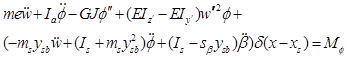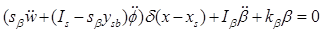(1)

where m is the mass per unit length, e is the distance from elastic axis to the center of gravity, EI and GJ are bending rigidity and torsional rigidity respectively, Ia is the mass moment of inertia, ms is the mass of store, Is is the moment of inertia of store with respect to the center of gravity,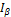is the moment of inertia of store to point B,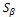is the static moment of mass to point B of store, ysb is the distance from the center of gravity of the store to elastic axis, xs is the span wise location of store and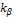is the wing-store joint rigidity.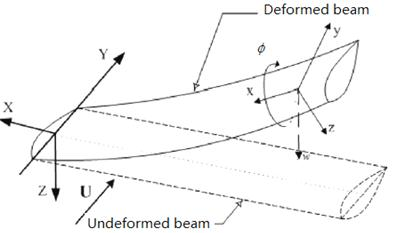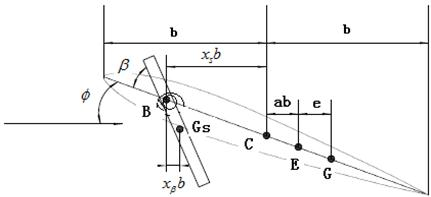Fig. 1. The model of wing-store system.

The unsteady aerodynamic forces can be written as formula (2) using the Wagner function in the Duhamel integral form,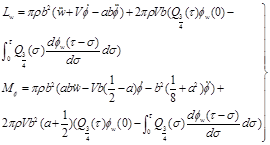(2)

where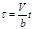is the non-dimensional time, V is the flow speed, b is semi-chord length, a is the non-dimensional distance from the elastic axle to the middle point of chord,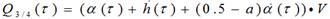,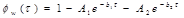with A1=0.165, A2=0.335, b1=0.0455, b2=0.3.

To avoid integrals terms in the state space representation,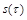is introduced as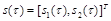,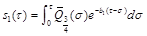,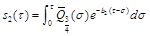The state vector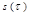satisfy the following differential equation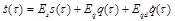(3)

where,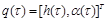,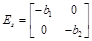,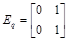,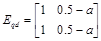.

The equations are discretized by Galerkin’s method and reorganized by non-dimensional parameters. The non-dimensional aeroelastic equations can be written as,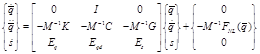(4)

Where,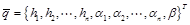is the displacement vector; M,C,K,G are mass, damping, rigidity and state vector matrix respectively.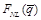is the nonlinear vector with respect to the displacement vector.

3. Flutter Boundary Analysis

The parameters of the wing are shown in table 1. The parameters of store are variables for the flutter characteristic study.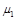is the mass ratio of store to wing.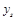is the non-dimensional chord wise location of store.

Table 1. Parameters of Wing.

 parameter value L (m) 1.2192 b (m) 0.1016 EI (N m2) 403.76 GJ (N m2) 198.58 m (kg m-1) 1.2942 Ia (kg m) 0.0036 Elastic axis (% chord) 43.70% CG (% chord) 45.40%

We first study the effect of location and wing-store rigidity on the critical flutter speed. Nonlinear terms are ignored in this case study.

The effect of span wise location of store on critical flutter speed is shown in Fig. 2. It should be pointed out that such results cannot be obtained from two dimensional model. The different parameter sets of store shown in figure 2 are as follows:

data1—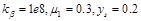;

data2---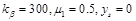data3--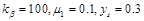;

data4--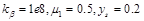.

As the location of store moves from wing root to wing tip, the critical flutter speed decreases first and then increases. When the store is located at wing tip, the critical flutter speed is the largest, while the smallest critical flutter speed usually occurs when the store location is at 30%-50% of the span length.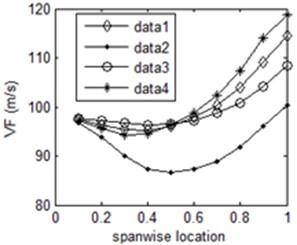Fig. 2. Critical flutter speed vs span wise location.

The effect of chord wise location of store on critical flutter speed is shown in Fig. 3. The different parameter sets of store in Fig. 3 are as follows:

data1—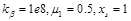;

data2---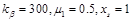;

data3---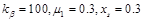;

data4--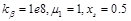;

data5--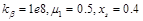;

data6--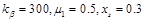.

It can be found from the two figures that the largest critical flutter speed occurs at about 40% semi-chord when the store is located close to the wing tip. If the store is near the wing root, the more forward the chord wise location is, the larger the critical flutter speed is.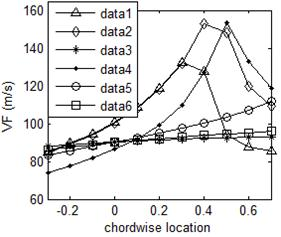Fig. 3. The critical flutter speed vs chord wise location.

The flutter boundaries in terms of flutter frequency are shown in Fig. 4. Here,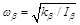, and the value of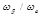is between 0 and 1.2. It can be seen from fig. 4 that the store parameters significantly influence the flutter boundary. But in general, the increasing trend of critical flutter speed with respect to increasing frequency is unchanged for a number of parameter sets. That is to say, if the joint has freeplay, the critical flutter speed will decrease. It should also be noticed that under some cases, the flutter frequency may change abruptly as the joint rigidity increases. This implies the motion frequency could change from high order frequency to lower order.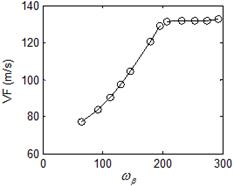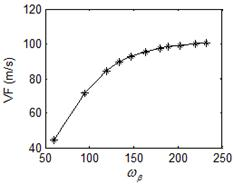(a)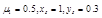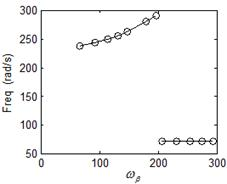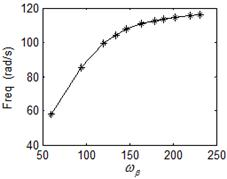(b)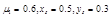Fig. 4. Flutter boundary with respect to frequency.

4. Buckling Boundary Analysis

Buckling is the static instability phenomenon of structures. By only keeping the time invariant terms of the motion equation, the static equations of the system can be written as,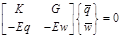(5)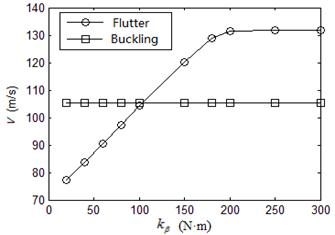Fig. 5. Flutter and buckling boundary of system.

The buckling boundary can be obtained by computing the load that satisfy zero determinant of the coefficient matrix of Equation (5). Here the first bending and torsional mode are used to calculate the analytic solutions. The results indicate the buckling boundary is independence of the parameters of store. The flow speed is 105.2m/s. By setting the parameters of store as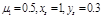, the flutter boundary and buckling boundary are calculated using the first three bending and torsional modes, which are shown in Fig. 5. It can be seen the numerical value of critical speed is 105.4m/s, which agrees well with the analytic solution. For this configuration of store, the critical joint rigidity is 100. Under this value, flutter will occur first in the system and above this value, buckling will occur first.

5. Nonlinear Responses

Letting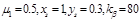, the system response with geometric nonlinearity of wing with store system is investigated. Bifurcation diagram of torsion angle at wing tip is shown in Fig. 6. The initial conditions is y0(8,1)=0.005. Phase portraits and Poincare maps of torsion angle at wingtip under different speeds are shown in Fig. 7. It can be seen that the torsion angle motion at wingtip evolves from limit-cycle oscillation, to quasi-periodical oscillation, and eventually chaos and periodic motions.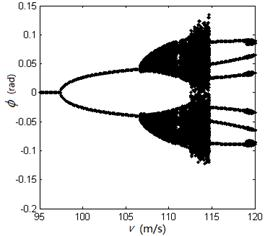Fig. 6. Bifurcation diagram of torsion angle at wingtip.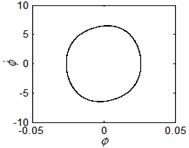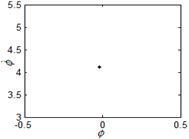(a)V=100m/s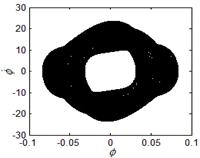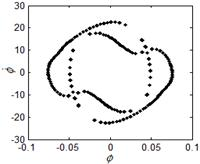(b) V=110m/s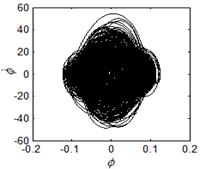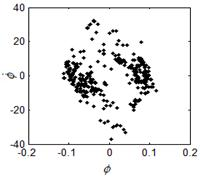(c) V=114m/s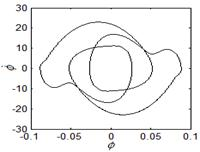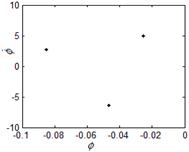(d) V=116m/s

Fig. 7. Phase portraits and Poincare maps for torsion angle of wingtip under different speeds.

To further study the complex dynamics of the wing-store system. We carried out intensive numerical simulations with the joint rigidity as changing variable. The response boundaries in terms of system parameters are plotted in figure 8. The motion pattern of each section labelled in the figure are explained as follows: section A is the convergent response; section B is the single stable limit cycle oscillation; section C is the buckling and finally section D is with complex motion that could be quasi-periodic, multi-periodic or even chaos.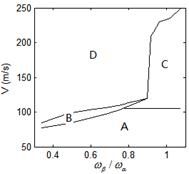Fig. 8. Responds boundary of the system.

6. Conclusion

This paper develops the dynamical model of long straight wing with store. The aeroelastic stability is numerically studied in terms of store parameters. The main conclusions are listed as follows:

(1)  Higher critical flutter velocity can be achieved by locating the store closer to wing tip;

(2)  Maximum flutter velocity occurs when the store is located at wing tip and its chord wise location is about 40% semi- chord ahead of the elastic axis;

(3)  Sudden change of flutter frequency may occur when the wing-store joint rigidity increases. The frequency changes from higher order to lower order;

(4)  The system buckling boundary is independent of store parameters. Flutter occurs first with small joint rigidity while buckling occurs first when the rigidity is large;

(5)  Geometric nonlinearity could result in complex system motions like limit cycle oscillation, quasi-periodic motion and chaos.

References

1. Yang Y R. KBM method of analyzing limit cycle flutter of a wing with an external store an d comparison with a wind tunnel test [J]. Journal of Sound and Vibration, 1995,187(2): 271-280.
2. Yang Zhichun, Zhao Lingcheng. The effects of pylon stiffness on the flutter of wing/store system[J].Chinese Journal of Applied Mechanics, 1993,6:1-7.
3. Zhang Jian, Xiang Jinwu. Stability of high-aspect-ratio flexible wings loaded by a lateral follower force[J].Acta Aeronautica and Astronautica Sinica,2010,31(11):2115-2122.
4. WANG Ganglin,XIE Changchuan. Flutter analysis with the thrust effects of engine under wing[J]. Aeronautical Science and Technology, 2014,25(06):22-27.
5. Xu Jun, Ma Xiaoping. Flutter analysis of a high-aspect-ratio wing with external store[J]. Mechanical Science and Technology for Aerospace Engineering,2015,34(4):636-640.
6. Y.M. Chen, J.K.Liu, G.Meng. An incremental method for limit cycle oscillations of an airfoil with an external store[J]. International Journal of Non-Linear Mechanics 47 (2012) 75–83.
7. S.A. Fazelzadeh, A.Mazidi,H.Kalantari. Bending-torsional flutter of wings with an attached mass subjected to a follower force[J]. Journal of Sound and Vibration 323 (2009) 148–162.
8. Chakradhar Byreddy, Ramana V. Grandhi2, and Philip Beran. Dynamic Aeroelastic Instabilities of an Aircraft Wing with Underwing Store in Transonic Regime[J]. Journal of Aerospace Engineering, Vol. 18, No. 4, October 1,2005,206–214.
9. D.M. Tang, E.H. Dowell. Effects of geometric structural nonlinearity on flutter and limit cycle oscillations of high-aspect-ratio wings[J]. Journal of Fluids and Structures 19 (2004) 291–306.
10. A. Mazidi and S. A. Fazelzadeh. Flutter of a Swept Aircraft Wing with a Powered Engine[J]. Journal of Aerospace Engineering, Vol. 23, No. 4, October 1, 2010,243–250.
11. Xu Jun, Ma Xiaoping. Flutter analysis of a high-aspect-ratio wing with external store[J]. Mechanical Science and Technology for Aerospace Engineering, 2015, 34(4):636-640.

 Contents 1. 2. 3. 4. 5. 6.
Article ToolsAbstractPDF(1370K)